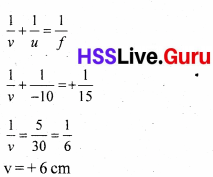# Kerala Syllabus 10th Standard Physics Solutions Chapter 4 Reflection of Light

## Kerala State Syllabus 10th Standard Physics Solutions Chapter 4 Reflection of Light

### Reflection of Light Text Book Questions and Answers

Text Book Page No. 80

Sslc Physics Chapter 4 Kerala Syllabus Question 1.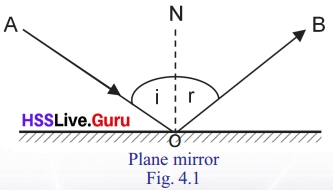→ Which is the incident ray?
AO

→ Which is the reflected ray?
QB

→ Is there any relation between the angle of incidence and the angle of reflection?
The angle of incidence and angle of reflection are equal.

→ Are the incident ray, reflected ray and normal to the mirror at the point of incidence in the different planes?
In the same plane.

Reflection Of Light Class 10 Kerala Syllabus Question 2.→ What difference is seen between the surfaces of the two objects?
In the first figure the surface is smooth, in the second figure surface is rough.

→ Fig. 4.2(b) are the rays of light travelling parallel after reflection?
No. When light falls on a rough surface, it undergoes an irregular reflection. This is scattered reflection.

Reflection of Light Class 10 Question 3.
In Fig. 4.2(a) regular reflection is depicted. Can you give a definition for such reflections by observing the figure?
When light falls on a smooth surface, it undergoes an regular reflection, the rays of light travelling parallel after reflection This is regular reflection.

Text Book Page No. 81

Sslc Physics Chapter 4 Notes Kerala Syllabus Question 4.
Record in your science diary the following features about the images formed here.→ The distance from the mirror to the object and the image from the mirror.

→ Is the image real or virtual?

→ The size of the image.
Same size as object.

Sslc Physics Reflection Of Light Kerala Syllabus Question 5.
How many images can we see at a time busying two mirrors?
The number of images changes accordance with the relation between the angle between the mirrors.

Text Book Page No. 82

Sslc Physics Chapter 4 Reflection Of Light Kerala Syllabus Question 6.
Table 4.1.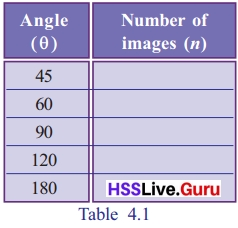Angle (0) Number of images (n) 45 7 60 5 90 3 120 2 180 1

→ How many images can be seen when viewed from A and B?
3

→ What if viewed from other positions in between the mirrors?
3

→ How much is the angle between the mirrors?
90°

→ What is the relation between the angle between the mirrors and the number of images?
Number of images = $$n=\frac{360}{\theta}-1$$

Class 10 Physics Chapter 4 Reflection Of Light Kerala Syllabus Question 7.
Table 4.2.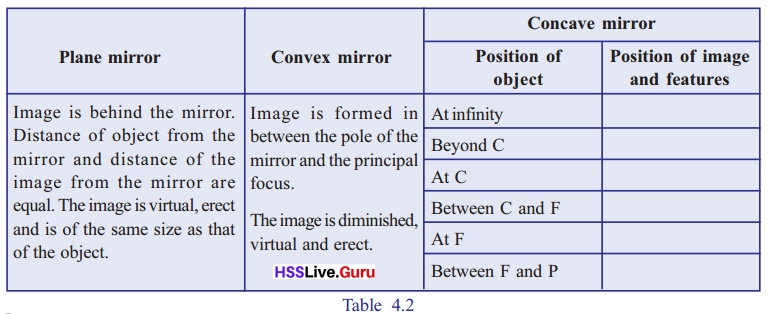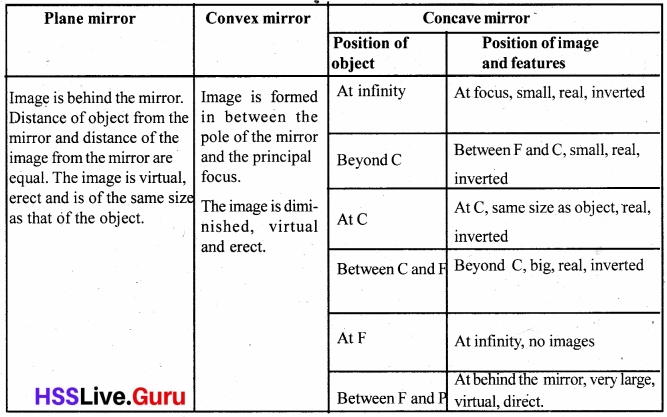Text Book Page No. 83

Class 10 Physics Chapter 4 Kerala Syllabus Question 8.Text Book Page No. 84

Sslc Physics Chapter 4 Solutions Kerala Syllabus Question 9.
Draw a straight line on a table as shown in the figure. At one end of the line, place a concave mirror of focal length 20 cm. Mark principal focus (F) and center of curvature (C) on the line.

Fix a burning candle on the principal focus in such a way that it is at a slight distance from the center of curvature. Arrange a screen in such a way that a clear image is obtained on the screen.

→ What is the position and features of the image?
Between F and C, small, real, inverted.

→ Observe the change in position of image and features on changing the position of the candlePhysics Chapter 4 Class 10 Kerala Syllabus Question 10.
Table 4.4.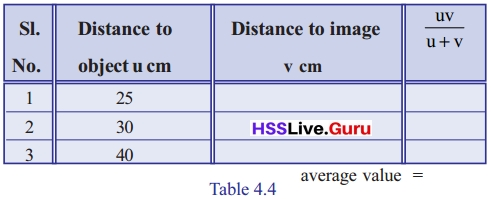Physics Class 10 Chapter 4 Kerala Syllabus Question 11.
Aren’t the focal length of $$\frac{u v}{u+v}$$ the mirror and the average value of obtained from the table the same?
Yes

Text Book Page No. 85

Sslc Physics Chapter 4 Reflection Of Light Notes Kerala Syllabus Question 12.
Record the measurements shown in the figure (4.6) using the New Cartesian Sign Convention.→ Distance to the object from the mirror (u) =
Negative.

→ Distance to the image from the mirror (v) =
Negative.

→ Height of object (OB) =
Positive.

→ Height of image (IM) =
Negative.

Text Book Page No. 86

Kerala Syllabus 10th Standard Physics Chapter 4 Question 13.
The given figure shows the image formation by a concave mirror. Analyse the figure and write down different measures using New Cartesian Sign Convention.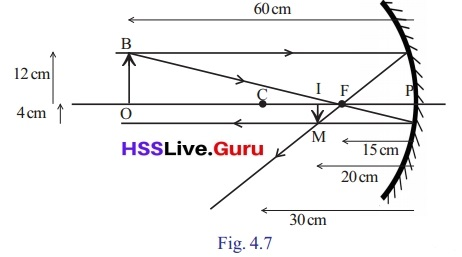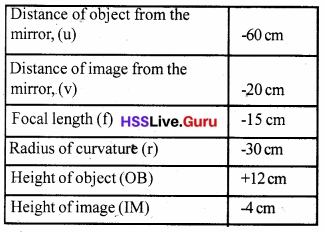Sslc Physics Chapter 4 Notes Pdf Kerala Syllabus Question 14.
An object is placed in front of a concave mirror 20 cm away from it. If its focal length is 40 cm, locate the position of image and its nature.
f = – 40 cm, u = – 20 cm
f = $$\frac { uv }{ u+v }$$
– 40 = $$\frac { – 20 }{ – 20 + v }$$
– 40 ( – 20 + v ) = – 20 v
– 20 + v = $$\frac { – 20 }{ – 40 }$$ = $$\frac { 2 }{ v }$$
– 20 = [/latex] = $$\frac { v }{ 2 }$$ – v = – $$\frac { v }{ 2 }$$
∴ – $$\frac { v }{ 2 }$$ = – 20
v = 40 cm
Features of image:
At behind the mirror, very large, virtual, direct.

10th Physics Chapter 4 Kerala Syllabus Question 15.
Find out the height of object (ho), height of image (hi), position of object(u) and position of image (v) using New Cartesian Sign Convention and tabulate them. (The height of image hi can be directly measured by fixing a graph paper on the screen).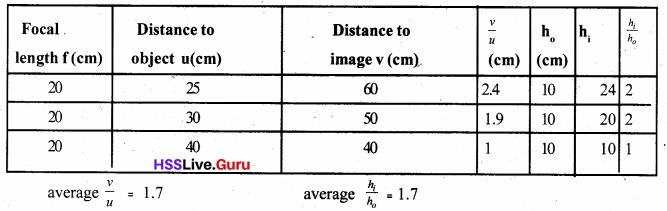Text Book Page No. 87

Physics Class 10 Chapter 4 Kerala Syllabus Question 16.
$$\frac{h_{i}}{h_{o}}$$ is magnification. Does it have any relational with the value of $$\frac{v}{u}$$ ?
$$m=\frac{-h_{i}}{h_{o}}=\frac{-v}{u}$$

Text Book Page No. 88

Question 17.
An object is placed 8 cm away in front of a concave mirror of focal length 5 cm. Find out the position of image and magnification. Find out whether the image is inverted or erect by drawing the ray diagram on a graph paper.
f = – 5 cm, u = – 8 cmFeatures of images : Beyond C, big, real, inverted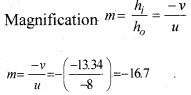Question 18.
What are the features of an image that is obtained from magnification?

• When magnification is 1, the size of the image and the size of the object are equal.
• When magnification is more than 1, the size of the image is greater than the size of the object.
• When magnification is less than 1, the size of the image is smaller than the size of the object.
• When the magnification is positive, image is real and inverted.
• When the magnification is negative, image is virtual and erect.

Question 19.
Observe the given figures and complete the table using New Cartesian Sign Convention.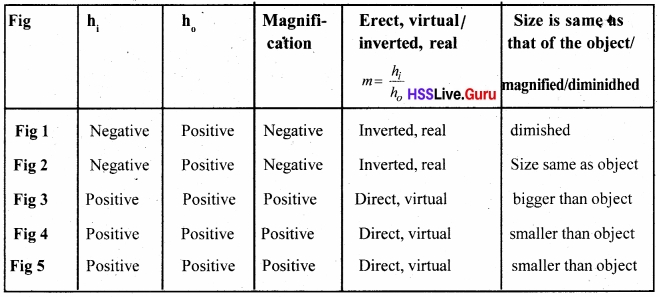Text Book Rage No. 89

Question 20.From the above table, find out which mirror always gives an erect and diminished image and write it down.
The image formed by a convex mirror is always erect and diminished.

Question 21.
Why it is written on rearview mirrors that “Objects in the mirror are closer than they appear”.
The image formed by a convex mirror is always erect and diminished. Hence the driver who sees the image of vehicles on the mirror develops a feeling that the vehicles coming from behind are at a greater distance. This may turn out to be dangerous.

### Reflection of Light Let Us Assess

Question 1.
A dental doctor uses a mirror of focal length 8 cm. To see the teeth clearly what should be the maximum distance between the teeth and the mirror? Justify your answer. Which type of mirror has been used by the doctor?
The dental doctor uses a concave mirror. Effect and enlarged image can be obtained using the mirror. Such images are formed when the object is kept in between the main focus and pole of a concave mirror. So the minimum distance between the mirror and the teeth must be in 8 cm to view the teeth clearly.

Question 2.
Imagine that a spherical mirror gives an image magnified 5 times at a distance 5 m. If so determine whether the mirror is concave or convex. How much will be the focal length of the mirror?Image formed is larger than the object, so the used mirror is concave.

Question 3.
A motorcyclist observes a car coining from behind with a magnification 1/6. If the actual distance between the car and the bike is 30 m calculate the radius of curvature of the mirror.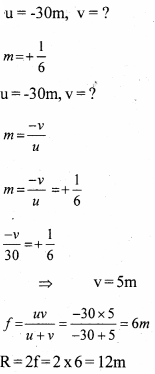Question 4.
A shaving mirror of focal length 72 cm is kept in a beauty clinic. A man uses it standing 18 cm away from the mirror. At what distance will the image be formed? Is the image real or virtual? What is the magnification of the image?
f =-72 cm, u = -18cm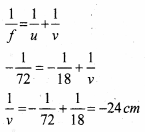Image formed on the 24 cm away from the mirror. Virtual image.
Magnification.
m = $$\frac{-v}{u}=-\frac{24}{18}$$ = – 1.33
This is a concave mirror.

Question 5.
Wrap a rubber ball of diameter 12 cm completely with an aluminum foil and make the surfaces smooth. Where will be the image of an object kept 12 cm away from the center of the ball? Is the image real or virtual?
Surface of a rubber ball is similar to a convex mirror.
Diameter = 12 cm
2f = R
f = $$\frac { R }{ 2 }$$ = $$\frac { 6 }{ 2 }$$ = 3 cm
u = $$\frac { 12 }{ 2 }$$ = 6 cmMirror is a convex, so image is virtual.

Question 6.
We are able to read a book since light falling on a surface gets reflected from the book and reaches the eye. But on such occasions, we cannot see our images like that from a mirror. Explain why?
Irregular reflection takes place in the book. So images not formed.

Question 7.
Is the image formed by a plane mirror real or virtual? Write an instance when such a mirror gives an inverted image.
Image formed by a plane mirror is always direct and virtual. The image will be at the same distance at the object.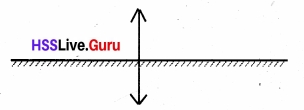If the objects placed erect as shown in the figure then the image will be inverted.

### Reflection of Light Exam Oriented Questions and Answers

Very Short Answer Type Questions (Score 1)

Question 1.
1. Classify the following statements as to those related to concave mirrors and convex mirrors and tabulate them accordingly.
a. to view the face.
b. as makeup mirror.
c. as reviewer mirrors in vehicles.
d. in solar concentration.
e. in periscopes.
f. as shaving mirror.
Concave mirror:

• In solar concentration.
• Makeup mirror.
• Shaving mirror.

Convex mirror:

• In rearview mirrors of vehicles.
• In Searchlights.

Plane mirror:

• In periscopes.
• To see face.

Question 2.
Find out the relation and All in the blanks.
Small, virtual and direct images:
Convex mirror.
Size same as object, virtual and direct image :………………
Plane mirror.

Question 3.
Which mirror gives rectifiable whatever the distance between the object and mirror is
i. Plane mirror.
ii. Convex mirror.
iii. Concave mirror.
iv. Plane or convex mirror.
Plane or convex mirror.

Question 4.
Relation between angle of incidence (i) and angle of reflection (r) of light is………..
i. i > r
ii. i < r
iii. i = r
iv. None of these
iii. i = r

Question 5.
Find the odd one in the group.
More scattered reflection, virtual image, direct image, large image
Large image.

Very Short Answer Type Questions (Score 2)

Question 6.
Radius of curvature of a concave mirror is 24 cm. Then find the focal length of the mirror?
R = 24 cm
f = $$\frac{R}{2}=\frac{24}{2}$$ = 12 cm

Question 7.
Find the radius of curvature of a convex mirror whose focal length is 0.6 m.
f = $$\frac{\mathrm{R}}{2}$$
∴ R=2f
0.6 = $$\frac{R}{2}$$
R = 2 × 0.6 = 1.2 m

Question 8.
Write the characteristics of the image formed by an object placed at the center of curvature of a concave mirror?
Position: At the center of curvature at the same side
Size: Same as the size of the object
Nature: Real, Inverted

Question 9.
i. Which are the different types of mirror?
ii. What are the peculiarities are images obtained in the face mirror?
i. Convex, concave and plane mirror.
ii. Image will be formed behind the mirror and will be equal distance from the mirror to the object. It will be direct and virtual.

Question 10.
State whether the below-given statement are true or false. If false correct them.
i. Real images are always produced by concave mirror.
ii. The image formed from a convex mirror is direct and enlarged.
i. False. If the position of object is between focus and pole, virtual image is produced by concave mirror.
ii.False. The image formed by convex mirror will be smaller than the object and erect.

Very Short Answer Type Questions (Score 3)

Question 11.
Write three differences of real image and virtual image which is made by spherical mirrors.
Real images:

• Inverted.
• Can be shown on a screen.
• Can measure the length of the image and distance to the image.

Virtual images:

• Cannot be taken on a screen.
• Not able to be measured direct.

Question 12.
Write the uses of concave mirror?

• Used as a shaving mirror.
• Used as a makeup mirror.
• Doctors used as head mirrors.
• On film projectors.

Question 13.a. Examine the figure and find the magnification.
b. What is the height of the object if height of the image is 4 cm when the object is placed on the same position in front of the mirror.
a. Magnification = $$=\frac{\mathrm{hi}}{\mathrm{ho}}=\frac{– 10}{5}=– 2$$Question 14.
Write the uses of convex mirror?

• As reflectors in street lights.
• As rear view mirrors in vehicles.
• In searchlights.

Question 15.
Examine the position of the object given in the figure and table the following peculiarities.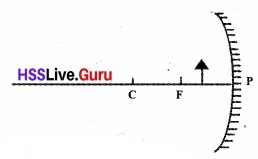a. Position of image.
b. Size of image.
c. Features of image.
a.Behind the mirror.
b.Larger than obj etc.
c. Direct, virtual.

Question 16.
When an object of height 4 cm is placed in front of a concave mirror an image of height 8 cm is formed. Find the magnification.
Magnification
hi = 4 cm, ho =  – 8 cm
Magnification m = $$\frac { hi }{ h0 }$$ = $$\frac { –8 }{ 4 }$$ = – 2

Very Short Answer Type Questions (Score 4)

Question 17.
Complete the table.a. Passes through principal focus.
b.Seem to come from the principal focus.
c, d. Returns parallel to the principal axis.
e, f. Returns through the same path.
g, h. Reflects in the same angle of incident ray.

Question 18.
Complete the ray diagram.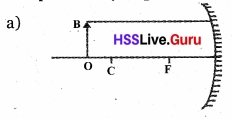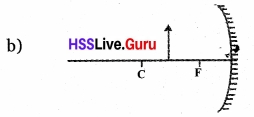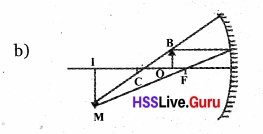Question 19.
Complete the table.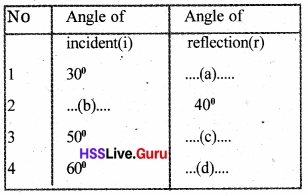a. 30°
b. 40°
c. 50°
d. 60°

Question 20.
An object of 5 cm is kept in front of a convex mirror having radius of curvature 30 cm at a distance of 10 cm. Calculate the position, size, and feature of object.
Focal length = $$\frac{r}{2}=\frac{30}{2}$$ = + 15 cm